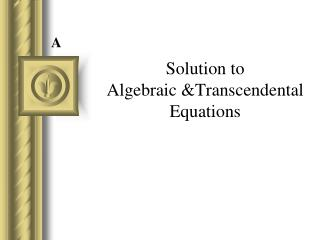Download PresentationSolution to Algebraic &Transcendental EquationsSolution to Algebraic &Transcendental Equations - PowerPoint PPT Presentation

Download PresentationSolution to Algebraic &Transcendental Equations
An Image/Link below is provided (as is) to download presentation

Download Policy: Content on the Website is provided to you AS IS for your information and personal use and may not be sold / licensed / shared on other websites without getting consent from its author. While downloading, if for some reason you are not able to download a presentation, the publisher may have deleted the file from their server.

- - - - - - - - - - - - - - - - - - - - - - - - - - - E N D - - - - - - - - - - - - - - - - - - - - - - - - - - -
Presentation Transcript

1. A Solution to Algebraic &Transcendental Equations

2. Algebraic functions The general form of an Algebraic function: fi = an i-th order polynomial. Example : f3 f2 f0 Polynomials are a simple class of algebraic function ai’s are constants.

3. Transcendental functions • A transcendental function is non-algebraic. • May include trigonometric, exponential, logarithmic functions • Examples:

4. Equation Solving • Given an approximate location (initial value) • find a single real root A Root Finding non-linear Single variable Open Methods Brackting Methods Iterative False- position Bisection Newton- Rapson Secant

5. A.1 Iterative method April 5, 2009

6. Simple Fixed-point Iteration • Rearrange the function so that x is on the left side of the equation: Now progressively estimate the value of x.

7. Problem • Find the root of f(x) = e-x – x • There is no exact or analytic solution • Numerical solution:

8. Iterative Solution • Start with a guess say x1=1, • Generate • x2=e-x1= e-1= 0.368 • x3=e-x2= e-0.368 = 0.692 • x4=e-x3= e-0.692=0.500 In general: After a few more iteration we will get

9. Iteration

10. Convergence Examples Convergent spiral pattern Convergent staircase pattern

11. Divergence Example Divergent spiral pattern Divergent staircase pattern

12. Existence of Root There exists one and only one root if L is Lipschitz constant,

13. Convergence? If x=a is a solution then, error reduces at each step i.e. iteration will converge If magnitude of 1st derivative at x=a is less than 1

14. Problem • Find a root near x=1.0 and x=2.0 • Solution: • Starting at x=1, x=0.292893 at 15th iteration • Starting at x=2, it will not converge • Why? Relate to g'(x)=x. for convergence g'(x) < 1 • Starting at x=1, x=1.707 at iteration 19 • Starting at x=2, x=1.707 at iteration 12 • Why? Relate to

15. A.2 Aitken’s Process

16. kth Order Convergence • Pervious iterative method has linear (1st order) convergence, since: • For kth order convergence we have: • Now consider a 2nd order method. Aitken’s 2 process

17. Aitken’s process • If  is a root of the equation i.e., =g() then, • Now if we use

18. Aitken’s process

19. Algorithm   guess_value; while (!   g()) { }

20. Why 2?